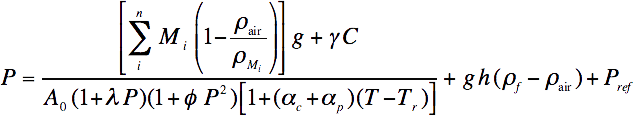The measurement of gravity is a critical factor when trying to achieve the highest degree of accuracy, or the lowest uncertainty. When using a deadweight tester or a piston gauge to measure pressure, it's important to consider the following:
• What is gravity?
• What effect does gravity have on the total uncertainty of a primary pressure measurement?
• Where do I find my local gravity?
• How can I be sure the reported gravity for my location is the actual gravity?
• What tools are available to incorporate gravity and other environmental factors into my measurements?
What is gravity?

Gravity is something taken for granted for most of human history, but in 1687, Isaac Newton formulated the law of gravitation, which states that a particle attracts any other particle in the universe with a force proportional to the product of their masses and inversely proportional to the square of the distance between them. This is the "classical" approach. In 1915, Albert Einstein published the general theory of relativity that describes gravity in terms of the curvature of space and time. The mathematics in Einstein's theory is much more complex than that of Newton's classical theory, but corresponds more precisely to observation.

Classical laws are sufficient when investigating the effect of gravity in the metrology of a deadweight tester. The universal gravitational constant (G) is the constant derived from the measurement of the force (F) between two masses (m1 and m2) at a distance (r). The equation for this relationship is F= G(m1·m2)/r2The local acceleration of gravity (g) is related to the gravitational constant by the equation g = (G · ME)/rE2 where ME is the mass of the earth, rE is the radius of the earth, and g is the acceleration of a mass falling in the gravity of the earth.

What effect does gravity have on the total uncertainty of a primary pressure measurement?

A deadweight tester is a primary pressure measurement standard that uses mass atop a piston-cylinder assembly to create a pressure under the piston. This pressure (P) is a direct result of the mass in gravity creating a force (F) acting on the cross sectional area (A) of the piston. Force divided by the area over which that force is applied is the definition of pressure, P=F/A. The force exerted by the mass is calculated by F=mg, where m is the mass loaded atop the piston and g is the acceleration of gravity at the location. Among other environmental conditions (temperature, air density, etc.), the value of the local acceleration of gravity is critical in the uncertainty of the pressure measurement.

A typical equation used to calculate the pressure created by a deadweight tester is:This equation takes into account all environmental factors and the physical components of the deadweight tester that affect the pressure generated. The uncertainty in all these factors - mass (M), air density (ρ), temperature (T), etc. - are statistically combined and form the total uncertainty in the generated pressure. The subject of this post is the local gravity (g). Notice that the local gravity term (g) appears twice in the equation. The first one, in the numerator, is used to calculate the force that results from the mass loaded on the piston. The last one is used to calculate the head pressure of the system. Accurate measurement of gravity is critical in the resultant pressure. In fact, there is about a 1:1 relationship between the uncertainty in the gravity (g) value and the uncertainty of the resulting pressure.Where do I find my local gravity?

Local gravity can be obtained from the National Oceanic and Atmospheric Association's National Geodetic Survey, or the PTB.de website by entering your longitude and latitude into the surface gravity prediction tools. The values obtained there are as accurate as the data from which they are derived and sometimes only vary from the actual gravity by less than 5 ppm, but that is not guaranteed. Dan Roman at NOAA says "the (NOAA) tool is an interpolator from a fixed database of gravity values. These spot gravity data can vary significantly in quality and distribution. For precision work, obtaining an onsite observation (with a gravimeter) is the best approach."

Typically the total uncertainty of a laboratory deadweight is below 35 ppm. Choosing the most accurate gravity value (g) is critical to the accuracy of a laboratory deadweight tester's resulting pressure measurement.

How can I be sure the reported gravity for my location is the actual gravity?

The only way to have a high degree of certainty in your local gravity value is to obtain an onsite observation. However, the decision to use a value for gravity obtained from a website or doing a local gravity measurement depends on the level of uncertainty that you can tolerate in your measurement. You may want to invest in a gravity measurement, but for a typical industrial deadweight tester with total uncertainty close to or above 80 ppm, it may not be necessary.

What tools are available to incorporate gravity and other environmental factors into my measurements?

Incorporating a gravity measurement and other environmental and physical quantities into the deadweight pressure equation can be a little daunting. Luckily, many deadweight testers are equipped with environmental sensors and there are instruments that incorporate all of the relevant factors into the equation to achieve minimal uncertainty in the measurement. The WIKA CPU6000 along with the CPB-CAL iPad® app calculates the masses required for a specific pressure and incorporates the pertinent environmental factors, including entry of local gravity (g), to achieve the highest accuracy in the pressure measurement.

## Author### Larry Mock

Marketing Communications Manager